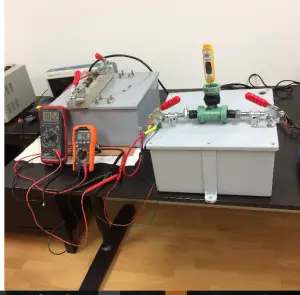# E-Cat QX Picture Posted in New Rossi-Gullstrom Paper (COP of 2000 reported with Calorimetry)

Thanks to Observer for pointing out a new paper that was published has been added to the Rossi-Gullstrom paper that was published on July 18 on Arxiv.org here. The title of the article is “Nucleon polarizability and long range strong force from σI=2 meson exchange potential”

It is a very involved theoretical paper for the most part. Here is the abstract.

“We present a theory for how nucleon polarizability may be used to extract energy from nucleons by means of special electromagnetic conditions.
Also a new theory for a long-range strong force is introduced by enhancing the role of the σI=2 meson in nucleon-nucleon potential obtained
through isospin mixed σ mesons. The novelty in the idea is to let an imaginary mass exchange particle be enhanced by absorbing only
one particle in an entangled state of two particles. The imaginary mass particle is not intendent to be free and contravance the law of physic; it is merely included as a binding exchange particle in a system with total positive invariant mass. In order to validate part of the theory, we introduce an experiment that in many ways have motivated this study”

At the end of the paper is an appendix which reports on two experiments, the first has already been reported in a previous paper, the second is one that has used calorimetry as the measurement system.

“The system is displayed in figure 5. In the figure, the yellow thermometer measures the temperature of the oil inside the heat exchanger. In the left in the figure there is two voltmeters that measure the mV of the current passing through the 1 Ohm brown resistance.

Calculations of the calorimetry made by the heat exchanger:
efficiency of the heat exchanger:10%
Primary heat exchange fluid: lubricant oil ( Shell mineral oil )
Characteristics of the lubricant oil: D = 0.9 Specific Heat: 0.5
Calorimetric data of the fluid: 0,5 Kcal/h = 0.57 Wh/h
Flow heating: 1.58 C / 1.8″ x 11 g
Resulting rating: 20 Wh/h
Energy input: V=0.1 R=1 Ohm → W=0.01
The COP of the system with the calorimetric measurement is substantially conciliable with the measurements made by the Wien’s equation and the Boltzmann equation.”

On the last page is an image of the experimental setups.